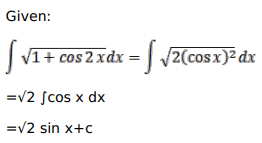# Solve this following

Question:

Mark $(\sqrt{ })$ against the correct answer in each of the following:

$\int \sqrt{1+\cos 2 x} d x=?$

A. $\sqrt{2} \cos x+C$

B. $\sqrt{2} \sin \mathrm{x}+\mathrm{C}$

C. $-\sqrt{2} \cos x+C$

D. $-\sqrt{2} \sin x+C$

Solution: